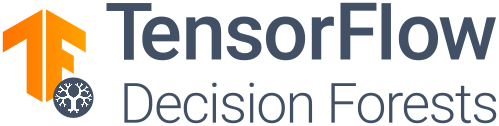New! Use Simple ML for Sheets to apply machine learning to the data in your Google Sheets
```# Install TF-DF
!pip install tensorflow tensorflow_decision_forests

import tensorflow_decision_forests as tfdf
import pandas as pd

# Load a dataset in a Pandas dataframe.

# Convert the dataset into a TensorFlow dataset.
train_ds = tfdf.keras.pd_dataframe_to_tf_dataset(train_df, label="my_label")
test_ds = tfdf.keras.pd_dataframe_to_tf_dataset(test_df, label="my_label")

# Train a Random Forest model.
model = tfdf.keras.RandomForestModel()
model.fit(train_ds)

# Summary of the model structure.
model.summary()

# Evaluate the model.
model.evaluate(test_ds)

# Export the model to a SavedModel.
model.save("project/model")
```TensorFlow Decision Forests (TF-DF) is a library to train, run and interpret decision forest models (e.g., Random Forests, Gradient Boosted Trees) in TensorFlow. TF-DF supports classification, regression, ranking and uplifting. It is available on Linux and Mac. Window users can use WSL+Linux.

TF-DF is powered by Yggdrasil Decision Forest (YDF), a library to train and use decision forests in c++, JavaScript, CLI, and Go. TF-DF models are compatible with YDF models, and vice versa.

Keywords: Decision Forests, TensorFlow, Random Forest, Gradient Boosted Trees, CART, model interpretation.

### Documentation & Resources

The following resources are available:

### Community

• TensorFlow forum
• TensorFlow Decision Forest on Github
• Yggdrasil Decision Forest on Github
• ### Contributing

Contributions to TensorFlow Decision Forests and Yggdrasil Decision Forests are welcome. If you want to contribute, make sure to review the developer manual.

[{ "type": "thumb-down", "id": "missingTheInformationINeed", "label":"Missing the information I need" },{ "type": "thumb-down", "id": "tooComplicatedTooManySteps", "label":"Too complicated / too many steps" },{ "type": "thumb-down", "id": "outOfDate", "label":"Out of date" },{ "type": "thumb-down", "id": "samplesCodeIssue", "label":"Samples / code issue" },{ "type": "thumb-down", "id": "otherDown", "label":"Other" }]
[{ "type": "thumb-up", "id": "easyToUnderstand", "label":"Easy to understand" },{ "type": "thumb-up", "id": "solvedMyProblem", "label":"Solved my problem" },{ "type": "thumb-up", "id": "otherUp", "label":"Other" }]